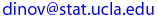## UCLA Statistics, Spring 2003

 Instructor: Ivo D. Dinov, Ph.D. Assistant Professor in Statistics, Research Scientist, Department of Neurology, UCLA School of Medicine E-mail:Teaching Assistan(s): C. Chang  E-mail: cchang@stat.ucla.edu

Textbook:  Probability and Statistics for Engineering and the Sciences, Jay Devore, Duxbury, 5th edition (2000).
Tentative schedule of topics to be covered
1. Introduction: What is Statistics? Population vs. Sample, Collection of data, random samples
2. Descriptive Statistics: Stem and leaf displays, Dotplots, Histograms, Numerical measures for center of distributions
3. Descriptive Statistics: Numerical measures for spread, Boxplot
4. Probability: Events, Axioms, Properties
5. Probability: Product Rules, Permutations, Combinations, Conditional probability, Independence, Bayes theorem
6. Discrete random variables: Probability distributions, Binomial, Negative Binomial, Hypergeometric, Poisson
7. Continuous random variables: Probability density function, Normal distribution
8. Continuous random variables: Normal approximation to the binomial, Exponential distribution
9. Two random variables: Joint, marginal and conditional distribution
10. Sampling: Simple random sampling, Sampling distribution, Central Limit Theorem (CLT)
11. Point estimation: Bias, Efficiency
12. Confidence intervals: CI for means and proportions on a single sample

Last modified on by.

Ivo D. Dinov, Ph.D., Departments of Statistics and Neurology, UCLA School of Medicine